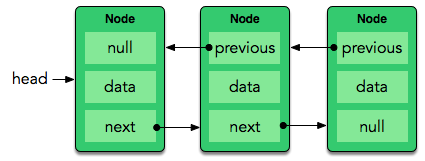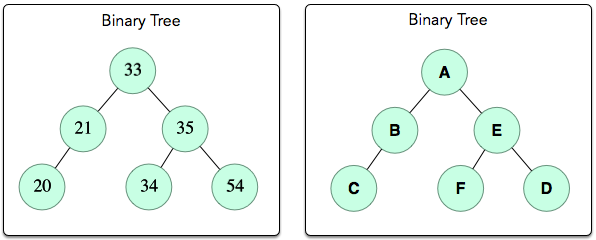## Cracking The Coding Interview

1. Who should join "Cracking the Coding Interview" workshop ?

1. Engineers {any stream}, MCA, BSc(CS/IT)
2. S/W developers
3. Web developers
4. Coders working with Small or Mid size companies
2. Why should I Join this Workshop ?

1. to learn practically core { + difficult } concepts of CS.
2. to solve challenges of companies like Google, Samsung, Amazon, etc...
3. to get invitation and participate in Top companies Coding contest { for lifetime }."Cracking the Coding Interview" workshop for 36 hrs is based on Amazon-best Selling bookThis workshop highlights and practically solves questions asked in coding interviews of Google, Amazon, Morgan Stanley, Goldman Sachs, Uber, OLX, Samsung and many more.

### Section 1: Data Structures

Arrays : 80 mins

This is a data structure that stores elements of the same type (generally), as a contiguous block of cells sequentially indexed from  to [n-1].

• Array_Coding_Problems_to_be_solved_in_Practical_class

ArrayList : 40 mins
While an array has a fixed size (meaning you can't add more elements to the end once it's full), an ArrayList (sometimes called a resizable or dynamic array) will grow as needed. We use an ArrayList when we want the benefits of an array (constant-time access to elements at particular indices) but don't know up front how many elements we'll need to store.

{in Java}Internally ArrayList are implemented over arrays.

• ArrayList_Coding_Problems_to_be_solved_in_Practical_class.Stack : 60 mins• Stack_Coding_Problems_to_be_solved_in_Practical_class

Queue : 60 mins

A queue is an abstract data type that uses a principle called First-In-First-Out (FIFO), meaning that the first object added to the queue must be the first object removed from it.

At minimum, any queue should be able to perform the following two operations:

• Enqueue: Add an object to the back of the line.
• Dequeue: Remove the object at the head of the line and return it; the element that was previously second in line is now at the head of the line.
• Queue_Coding_Problems_to_be_solved_in_Practical_class

Hashing : 60 mins

This refers to the process of converting a key into an index by performing some simple operation on the key. The data structure used to hold the Keys and its value is called Hash tables.

• Hashing_Coding_Problems_to_be_solved_in_Practical_class

Binary Tree : 90 mins• Binary_Tree_Coding_Problems_to_be_solved_in_Practical_class

Heaps : 90 mins

A heap is just what it sounds like — a pile of values organized into a binary tree-like structure adhering to some ordering property. When we add elements to a heap, we fill this tree-like structure from left to right, level by level. This makes heaps really easy to implement in an array, where the value for some index i's left child is located at index (2i+1) and the value for its right child is at index 2i+2(using zero-indexing). Here are the two most fundamental heap operations:

• add: Insert an element into the heap. You may also see this referred to as push.
• poll: Retrieve and remove the root element of the heap. You may also see this referred to as pop.
Tries : 90 mins

There are many algorithms and data structures to index and search strings inside a text, some of them are included in the standard libraries, but not all of them; the trie data structure is a good example of one that isn’t.

### Section 2: Algorithms

Bubble Sort : 40 mins

This is a very simple sorting algorithm. Because it's also very inefficient, Bubble Sort is not practical for real-world use and is generally only discussed in an academic context. The basic theory behind BubbleSort is that you take an array of integers and iterate through it; for each element at some index whose value is greater than the element at the index following it (i.e., index i+1 ), you must swap the two values. The act of swapping these values causes the larger, unsorted values to float to the back (like a bubble) of the data structure until they land in the correct location.

QuickSort and Comparator Interface {in Java} : 120 mins

The principle of the quick-sort algorithm is this:

1. Pick a "pivot" element.
2. "Partition" the array into 3 parts:
1. First part: all elements in this part is less than the pivot.
2. Second part: the pivot itself (only one element!)
3. Third part: all elements in this part is greater than or equal to the pivot.
3. Then, apply the quicksort algorithm to the first and the third part. (recursively)
Merge Sort and Counting Inversion Problem : 120 mins

Merge sort uses divide-and-conquer : Divide by finding the number q of the position midway between p and r. Do this step the same way we found the midpoint in binary search: add p and r, divide by 2, and round down.

Conquer by recursively sorting the subarrays in each of the two subproblems created by the divide step. That is, recursively sort the subarray array[p..q] and recursively sort the subarray array[q+1..r].

Combine by merging the two sorted subarrays back into the single sorted subarray array[p..r].

1. Counting_Inversion_Problem_13

Solving the Ice Cream Parlor problem : 120 mins

Each time Sunny and Johnny take a trip to the Ice Cream Parlor, they pool together money dollars for ice cream. On any given day, the parlor offers a line of n flavors. Each flavor,i , is numbered sequentially with a unique ID number from 1 to n and has a cost, costi, associated with it.

1. IceCream_Parlour_Problem_14

Concept of DFS 120 mins

Traversal means visiting all the nodes of a graph. Depth first traversal or Depth first Search is a recursive algorithm for searching all the vertices of a graph or tree data structure. For example we could use DFS to find all stations {nodes} reachable from a given source station. {say "How many stations can you reach from Dadar ?" - in your mind read stations as nodes }

1. Find_max_connected_cells_using_DFS_problem_15

Concept of BFS 120 mins

Traversal means visiting all the nodes of a graph. Breadth first traversal or Breadth first Search is a recursive algorithm for searching all the vertices of a graph or tree data structure. For example we could use BFS to find the shortest path from 1 point to another. {say "What shortest route could I take from Dadar to thane ?" }

1. Find_Shortest_path_using_BFS_problem_16

### Section 3: Techniques and "must know" CS concepts

Time Complexity : 60 mins

When discussing the time complexity of an algorithm, we use the positive integer n to represent the size of the data set it processes. To evaluate the actual algorithm, we must ignore machine-dependent constants (i.e., think about the number of instructions being executed, not about how fast a certain machine can execute them) and look at its growth rate as approaches (i.e., how does it grow as a function of time — especially as gets n large?)

Recursion : 40 mins

This is an extremely important algorithmic concept that involves splitting a problem into two parts: a base case and a recursive case. The problem is divided into smaller subproblems which are then solved recursively until such time as they are small enough and meet some base case; once the base case is met, the solutions for each subproblem are combined and their result is the answer to the entire problem.

{ Recursive Solution to Fibonacci gives the worst case. Best is Iterative method with memorization. For very large numbers use the 5th approach as below }

Note : There are many ways to solve the fibonacci problem

1. Iterative solution without memoization
2. Iterative solution with memorization
3. Recursive solution without memorization
4. Recursive solution with memorization
5. Recursive solution using formulas for F(2n) and F(2n+1) with or without memorization.

Recursive solution using formulas for F(2n) and F(2n+1) with or without memorization.

Solving Davis Staircase Problem using Recursion : 40 mins

Davis has s staircases in his house and he likes to climb each staircase , , or steps at a time. Being a very precocious child, he wonders how many ways there are to reach the top of the staircase.

Given the respective heights for each of the s staircases in his house, find and print the number of ways he can climb each staircase on a new line.

Dynamic Programming : 60 mins
Bit Manipulation: Lonely Integer : 40 mins

Consider an array of n integers,A=[a1,a2,... ai, .. an] , where all but one of the integers occur in pairs. In other words, every element in A occurs exactly twice except for one unique element.

Given A , find and print the unique element.Duration :

10 days { around 45 hrs }

carry laptops with jdk 8 installed

Fees :

₹ 9900*

{ * Special discounted fee of ₹ 9000/- if joining with Python Classroom Course }

{ Entire course is taken by Rocky Sir }

{ For Course contents scan this page top - to - bottom }

Where is this course conducted ?

Online Live class, Thane & Dadar Centers.

Whats-app No. :

9892544177